Courses

# Methods of Structural Analysis Civil Engineering (CE) Notes | EduRev

## GATE : Methods of Structural Analysis Civil Engineering (CE) Notes | EduRev

The document Methods of Structural Analysis Civil Engineering (CE) Notes | EduRev is a part of the GATE Course Structural Analysis II.
All you need of GATE at this link: GATE

Q.1 A portal frame shown in figure (not drawn to scale) has a hinge support at joint P and a roller support at joint R. A point load of 50 kN is acting at joint R in the horizontal direction. The flexural rigidity. El, of each member is 106 kNm2. Under the applied load, the horizontal displacement (in mm, round off to 1 decimal place) of joint R would be______.    [2019 : 2 Marks, Set-I]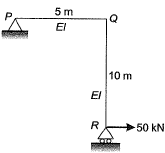Solution: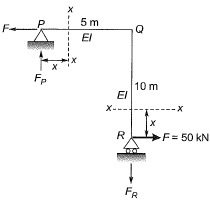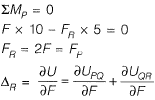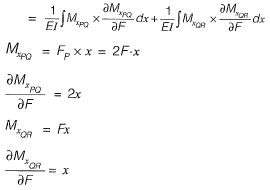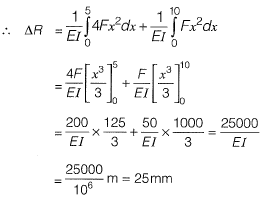Q.2 The rigid-jointed plane frame QRS shown in figure is subjected to load P at the joint R. Let the axial deformations in the frame be neglected. If the support S undergoes a settlement of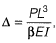the vertical reaction at the support S will be become zero when β is equal to    [2019 : 2 Marks, Set-I]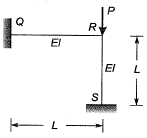(a) 7.5
(b) 3.0
(c) 48.0
(d) 0.1
Ans. (A)

Solution:
Assume 'R sinks by Δ.
Sway analysis: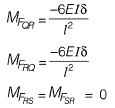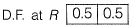End moment distribution,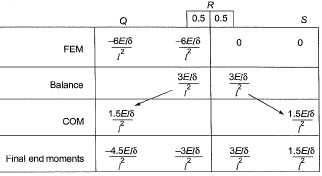Sway force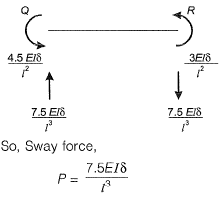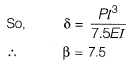Alternate method: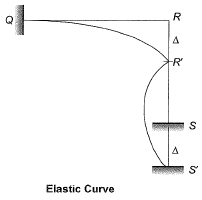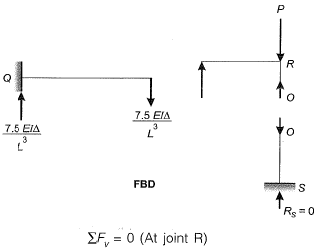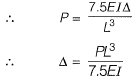Q.3 A prismatic beam P-Q-R of flexural rigidity El = 1 x 104 kNm2 is subjected to a moment of 180 kNm at Q as shown in the figure.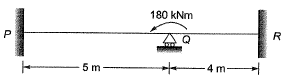The rotation at Q (in rad, up to two decimal places) is ________ .    [2018 : 2 Marks, Set-II]
Solution:

Method-1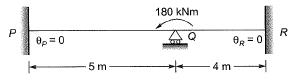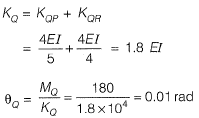Method-ll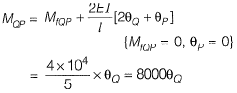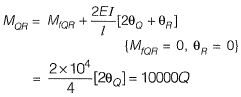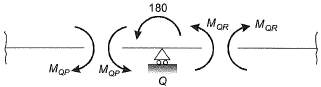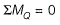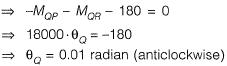Q.4 A vertical load of 10 kN acts on a hinge located at a distance of LI4 from the roller support Q of a beam of length L (see figure).    [2018 :1 Mark, Set-II]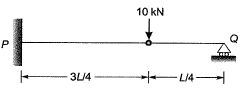The vertical reaction at support Q is
(a) 0.0 kN
(b) 2.5 kN
(c) 7.5 kN
(d) 10.0 kN
Ans.
(A)
Solution: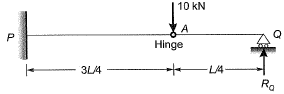Bending moment about hinge point A = 0
(consider the right hand side of A)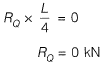Q.5 Consider the portal frame shown in the figure and assume the modulus of elasticity, E = 2.5 x 104 MPa and the moment of inertia, I = 8 x 108 mm4 for all the members of the frame.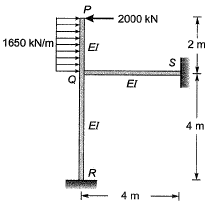The rotation (in degrees, up to one decimal place) at the rigid joint Q would b e _________ .    [2017 : 2 Marks, Set-II)
Solution:

Method-I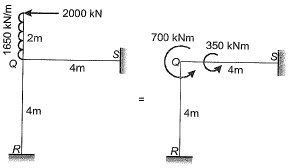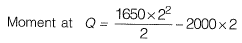= -700 kNm (ACW)
EI = 2.5 x 104 x 8 x 108
= 20 x 1012 Nmm2
= 20000 kNm2
As the two members QR and QS are identical, moment will be equally divided i.e., 350 kNm each.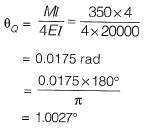Method-ll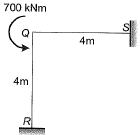Fixed end moment: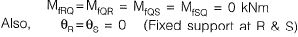Slope deflection equation: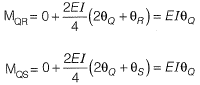Joint equilibrium equation at joint Q,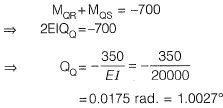Q.6 The value of M in the beam ABC shown in the figure is such that the joint B does not rotate.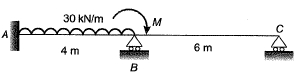The value of support reaction (in kN) at B should be equal to ________.    [2017 : 2 Marks, Set-I]
Solution:

Method-I
Fixed end moment: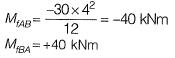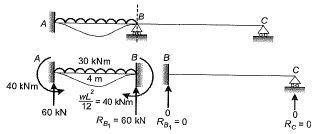By super position,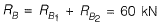Note : The value of 'M' shown in the figure,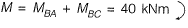Q.7 The portal frame shown in the figure is subjected to a uniformly distributed vertical load w(per unit length).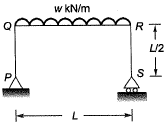The bending moment in the beam at the join ‘Q ’ is    [2016 : 2 Marks, Set-II]
(a) zero
(b)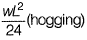(c)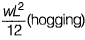(d)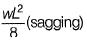Ans.
(A)
Solution: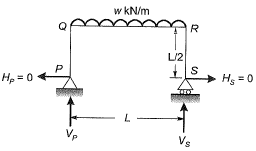As there is no horizontal force,
Hence,
HP = HS = 0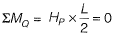∴ BM at Q = 0

Q.8 For the beam shown below, the value of the support moment M is ____ kN-m.    [2015 : 1 Mark, Set-I]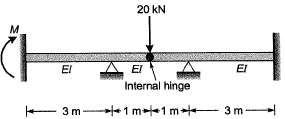Solution:

By symmetry we can consider two parts of beam separately with half the load.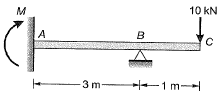Moment at joint B = 10 kNm
Carry over moment at joint A due to 10 kNm moment at propped end = 10/2= 5kN-m

Q.9 Considering the symmetry of a rigid frame as shown below, the magnitude of the bending moment (in kNm) at P (preferably using the moment distribution method) is    [2014 : 2 Marks, Set-II]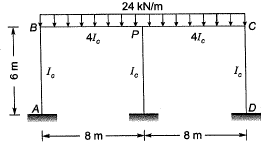(a) 170
(b) 172
(c) 176
(d) 178
Ans.
(C)
Solution:
As the frame and loading is symmetrical, θP= 0. So, joint Pcan be replaced by a fixed support as shown.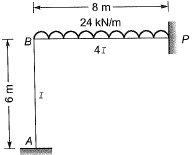Fixed end moment: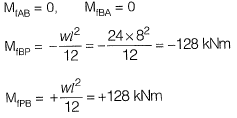Distribution factor: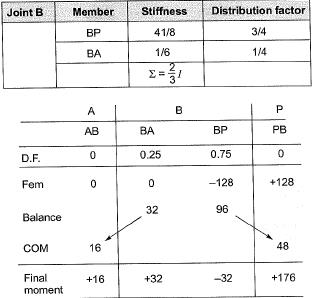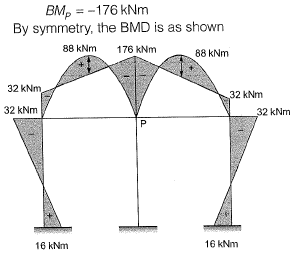Q.10 All members in the rigid-jointed frame shown are prismatic and have the same flexural stiffness EI. Find the magnitude of BM at Q (in kN-m) due to given loading.    [2013 : 2 Marks]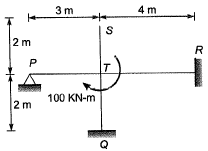Solution: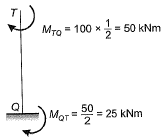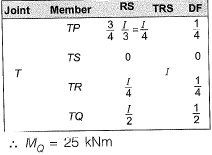Offer running on EduRev: Apply code STAYHOME200 to get INR 200 off on our premium plan EduRev Infinity!

## Structural Analysis II

109 docs|22 tests

,

,

,

,

,

,

,

,

,

,

,

,

,

,

,

,

,

,

,

,

,

;